11.4.2 Step 4: Rebalance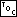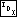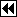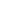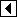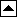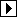```422. <Step 4: Rebalance after RTAVL insertion 422> =
if (y->rtavl_balance == -2)
{     <Step 4: Rebalance RTAVL tree after insertion to left 423>   }
else if (y->rtavl_balance == +2)
{     <Step 4: Rebalance RTAVL tree after insertion to right 424>   }
else   return &n->rtavl_data;

return &n->rtavl_data;
```

This code is included in 419.

The code to choose between the two subcases within the left-side and right-side rebalancing cases follows below. As usual during rebalancing, y is the node at which rebalancing occurs, x is its child on the same side as the inserted node, and cases are distinguished on the basis of x's balance factor:

```423. <Step 4: Rebalance RTAVL tree after insertion to left 423> =
struct rtavl_node *x = y->rtavl_link;
if (x->rtavl_balance == -1)
{     <Rebalance for - balance factor in RTAVL insertion in left subtree 425>   }
else   {     <Rebalance for + balance factor in RTAVL insertion in left subtree 427>   }
```

This code is included in 422.

```424. <Step 4: Rebalance RTAVL tree after insertion to right 424> =
struct rtavl_node *x = y->rtavl_link;
if (x->rtavl_balance == +1)
{     <Rebalance for + balance factor in RTAVL insertion in right subtree 426>   }
else   {     <Rebalance for - balance factor in RTAVL insertion in right subtree 428>   }
```

This code is included in 422.

##### Case 1: x has taller subtree on side of insertion

If node x's taller subtree is on the same side as the inserted node, then we perform a rotation at y in the opposite direction. That is, if the insertion occurred in the left subtree of y and x has a - balance factor, we rotate right at y, and if the insertion was to the right and x has a + balance factor, we rotate left at y. This changes the balance of both x and y to zero. None of this is a change from unthreaded or fully threaded rebalancing. The difference is in the handling of empty subtrees, that is, in the rotation itself (see RTBST Rotations).

Here is a diagram of left-side rebalancing for the interesting case where x has a right thread. Taken along with x's - balance factor, this means that n, the newly inserted node, must be x's left child. Therefore, subtree x has height 2, so y has no right child (because it has a -2 balance factor). This chain of logic means that we know exactly what the tree looks like in this particular subcase: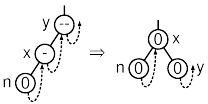```425. <Rebalance for - balance factor in RTAVL insertion in left subtree 425> =
w = x;
if (x->rtavl_rtag == RTAVL_THREAD)   {
x->rtavl_rtag = RTAVL_CHILD;
}
x->rtavl_balance = y->rtavl_balance = 0;
```

This code is included in 423.

Here is the diagram and code for the similar right-side case: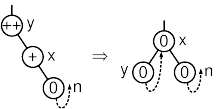```426. <Rebalance for + balance factor in RTAVL insertion in right subtree 426> =
w = x;
if (x->rtavl_link == NULL)   {
}
x->rtavl_balance = y->rtavl_balance = 0;
```

This code is included in 424.

##### Case 2: x has taller subtree on side opposite insertion

If node x's taller subtree is on the side opposite the newly inserted node, then we perform a double rotation: first rotate at x in the same direction as the inserted node, then in the opposite direction at y. This is the same as in a threaded or unthreaded tree, and indeed we can reuse much of the code.

The case where the details differ is, as usual, where threads or null child pointers are moved around. In the most extreme case for insertion to the left, where w is a leaf, we know that x has no left child and s no right child, and the situation looks like the diagram below before and after the rebalancing step: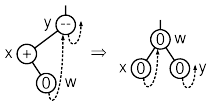```427. <Rebalance for + balance factor in RTAVL insertion in left subtree 427> =
<Rotate left at x then right at y in AVL tree; avl => rtavl 156>
if (x->rtavl_link == NULL)   {
}
if (w->rtavl_rtag == RTAVL_THREAD)   {
w->rtavl_rtag = RTAVL_CHILD;
}
```

This code is included in 423 and 442.

Here is the code and diagram for right-side insertion rebalancing: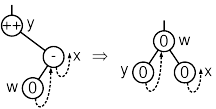```428. <Rebalance for - balance factor in RTAVL insertion in right subtree 428> =
<Rotate right at x then left at y in AVL tree; avl => rtavl 159>
if (y->rtavl_link == NULL)   {